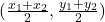## Describe and correct the error a student made in finding the midpoint cd with c(-4,5) and d(-1,-4).

Question

Describe and correct the error a student made in finding the midpoint cd with c(-4,5) and d(-1,-4).

in progress 0
5 months 2021-08-08T14:07:02+00:00 1 Answers 18 views 0

## Answers ( )

Step-by-step explanation:

The formula for finding the midpoint of two coordinates is expressed as;

M(X,Y) =Given the coordinates c(-4,5) and d(-1,-4), x1 = -4, y1 = 5, x2 = -1 and y2 = -4.

For the X coordinate of the midpoint

X = x1+x2/2

X = -4+(-1)/2

X = -4-1/2

X = -5/2

X = -2.5

Similarly for Y:

Y = y1+y2/2

Y = 5+(-4)/2

Y = 5-4/2

Y = 1/2

Y = 0.5

Hence the midpoint coordinate of C(-4,5) and D (-1,-4) is (-2.5, 0.5)